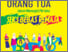## DIAGONALISASI MATRIKS ATAS RING KOMUTATIF DAN FIELD

OKTAVINASARI, AMALIA (2008) DIAGONALISASI MATRIKS ATAS RING KOMUTATIF DAN FIELD. Other thesis, University of Muhammadiyah Malang.Preview
Text
DIAGONALISASI_MATRIKS_ATAS_RING_KOMUTATIF_DAN_FIELD.pdf

## Abstract

Matrix is not a weird thing in math. Many things could be fulfilled by using matrix. Some matrix usage was to finished linear equation system, make analysis about economic with many variables easier. Matrix also could be fulfilled to reveal problem about diagonalization. In matrix could be diagonalyzed if there is a S matrix with invers so that , with D is a diagonal matrix. This research aimed to find out matrix which diagonalized matrix or commutative area and also to find diagonalyzed matrix in field. Matrix could be stated as matrix on commutative field when the element of the matrix were members of commutative field where commutative field was in-empty association with two binnary operation of summation and multiplication fulfilled two commutative group and semi-group with the close-characteristic , associative , and distributic characteristic . While for matrix could be said as matrix on field when the elements of the matrix was element from field where the field was a part of commutative area. Matrix to the commutative field could be diagonalyzed while matrix columns verctor which would diagonalyzed matrix formed from eigen verctors of the matrix ranged and linear free or formed basis. While field matrix could be diagonalyzed while vectors of matrix column which would diagonalyzed matrix formed from eigen vectors from the matrix were linear free.

Item Type: Thesis (Other) L Education > L Education (General) Faculty of Teacher Training and Education > Department of Mathematics Education (84202) Anggit Aldila 24 May 2012 05:48 24 May 2012 05:48 http://eprints.umm.ac.id/id/eprint/5864View Item